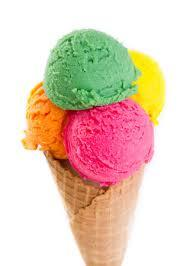# Strawberry 4769

They sold 4/7 strawberry ice cream and 36 servings of vanilla ice cream in the store. How many servings of ice cream did they sell that day?

n =  84

### Step-by-step explanation:

n = 4/7 n + 36

n = 4/7•n + 36

3n = 252

n = 84

Our simple equation calculator calculates it.Did you find an error or inaccuracy? Feel free to write us. Thank you!

Tips for related online calculators
Need help calculating sum, simplifying, or multiplying fractions? Try our fraction calculator.
Do you have a linear equation or system of equations and looking for its solution? Or do you have a quadratic equation?